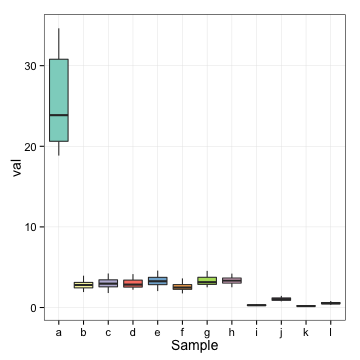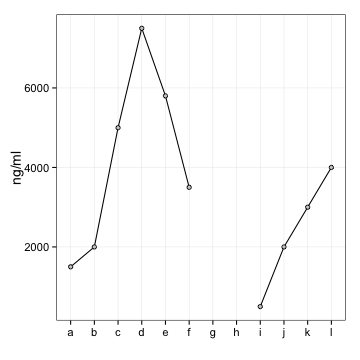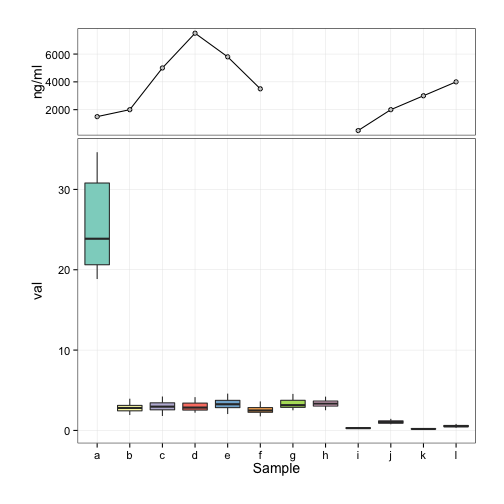# Aligning ggplot2 graphs on a page

May 22, 2014

Let’s say you have two graphs, a box plot and a line graph, with the same x axis and would like to have them aligned in a single two panel plot. Since the y axes are unrelated, we want to keep them as separate panels and avoid a confusing secondary y axis but we want the line graph to be smaller and sit on top of the box plot.

Some libraries we’re going to need

library(ggplot2)
library(gtable)

## Loading required package: grid

library(grid)


First, generate some made up data for the box plot

mv <- sqrt(c(25, runif(7, 2.5, 3.5), runif(4, 0, 1)))
mn <- length(mv)
ms <- letters[1:mn]
df <- NULL
for (i in 1:20) {
df <- rbind(df, data.frame(sample = ms, val = (mv + rnorm(mn, 0, 0.1 * mv))^2))
}
summary(df)

##      sample         val
##  a      : 20   Min.   : 0.12
##  b      : 20   1st Qu.: 0.78
##  c      : 20   Median : 2.63
##  d      : 20   Mean   : 4.08
##  e      : 20   3rd Qu.: 3.40
##  f      : 20   Max.   :34.63
##  (Other):120


Here is what the box plot should look like

p.box <- ggplot(df) +
geom_boxplot(aes(sample, val, fill = sample)) +
scale_fill_brewer(palette = "Set3") +
labs(x = "Sample", "Measure") +
theme_bw(14) +
theme(panel.grid.minor = element_blank(),
panel.border = element_rect(color = "black"),
legend.position = "none")
print(p.box)Figure 1: Box plot

And now the line plot data

drug.level <- data.frame(sample = factor(ms), ng = c(15, 20, 50, 75, 58, 35,
NA, NA, 5, 20, 30, 40) * 100)


And the plot itself

p.line <- ggplot(drug.level) +
geom_line(aes(sample, ng, group = 1)) +
geom_point(aes(sample, ng), pch = 21, fill = "grey80") +
labs(x = "", y = "ng/ml") +
theme_bw(14) +
theme(panel.grid.minor = element_blank(),
panel.border = element_rect(color = "black"),
legend.position = "none")
print(p.line)

## Warning: Removed 2 rows containing missing values (geom_point).Figure 2: Line plot

I purposefully set the scale so that the axis labels on the line plot are longer, which leads to unaligned plots when they are just stacked up. So we need to adjust the widths of the plots to be equal. In order for the plots to look nice, we also need to remove some margins and remove the axis labels on the line plot:

p.box <- p.box + theme(plot.margin = unit(c(-0.2, 1, 1, 1), "cm"))
p.line <- p.line + theme(axis.text.x = element_blank(),
axis.title.x = element_blank(),
axis.ticks.x = element_blank(),
plot.margin = unit(c(1, 1, -0.2, 1), "cm"))


We create grobs (grid objects) from the graphs

g.box <- ggplotGrob(p.box)
g.line <- ggplotGrob(p.line)

## Warning: Removed 2 rows containing missing values (geom_point).


Each of the grobs has a widhts vector that contains unit sized widths for the different parts of a plot. Parts 2-5 seem to be the ones we’re interested in. Find the maximum for each part across the plots and set the widths of all the plots to that maximum

maxwidths <- grid::unit.pmax(g.box$widths[2:5], g.line$widths[2:5])
g.box$widths[2:5] <- as.list(maxwidths) g.line$widths[2:5] <- as.list(maxwidths)


And then create a gtable (higher level interface to the grid system) with 2 rows, one of which is less tall than the other

g <- gtable_matrix(name = "demo",
grobs = matrix(list(g.line, g.box), nrow = 2),
widths = unit(7, "in"),
heights = unit(c(2, 5), "in"))


And draw the gtable

grid.newpage()
grid.draw(g)Figure 3: Combined plot